# OECD Gross pension replacement rates data analysis 6 - Linear Regression Analysis finds GDP does not matter for pension replacement rates.Created by Bing Image Creator

This post is following of the above test. In this post I will analyze relationship between replacement rates and per capita GDP.

So, I got per capita GDP data from OECD web site.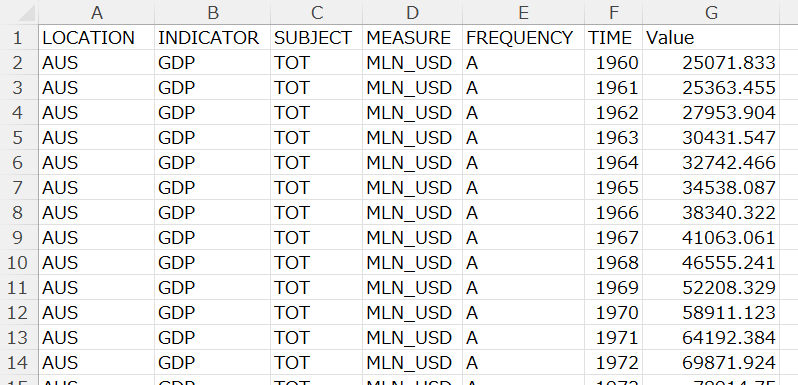I modified abve CVS file like below.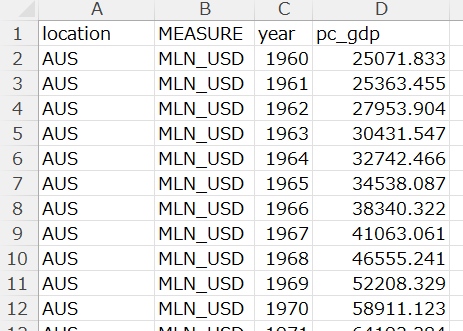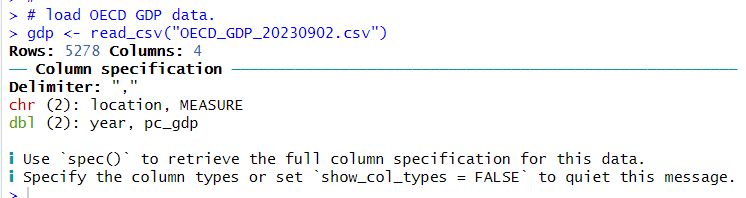SInce MEASURE has two values, one is MLN_USD and the other is USD_CAP, I converted "gdp" data frame to wide format.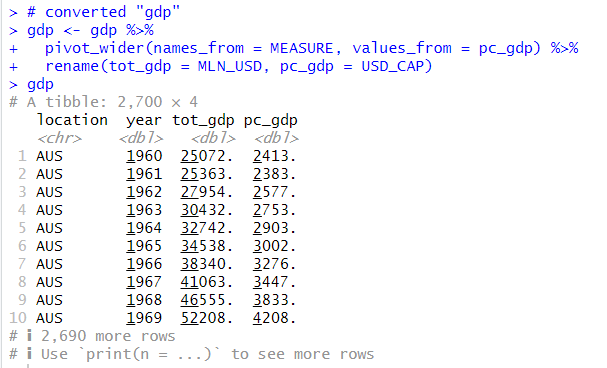All right, tot_gdp is total GDP in million USD, pc_gdp is per capita GDP in USD.

Let's merge "df" data frame and "gdp" data frame with inner_join() function.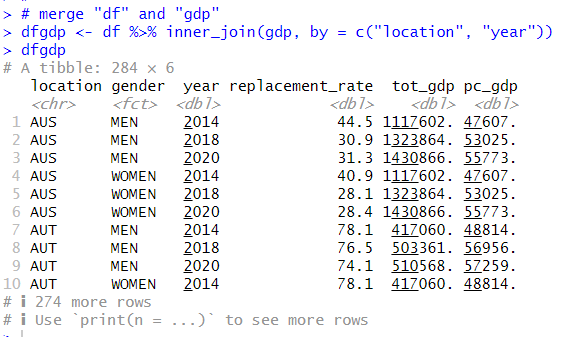I learned that there is not statistically significant differences between years, genders from the previous posts, so I avergae dfgdp by location.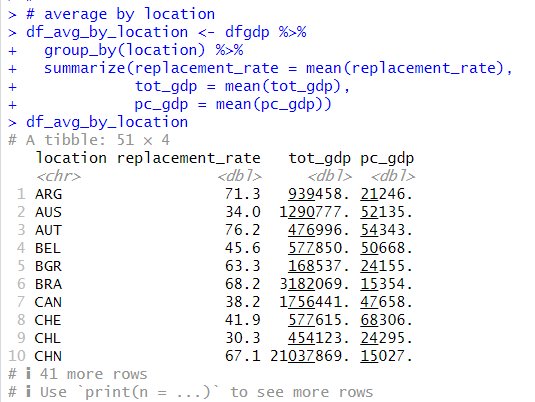Now, let's see scatter plots.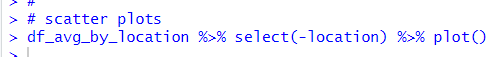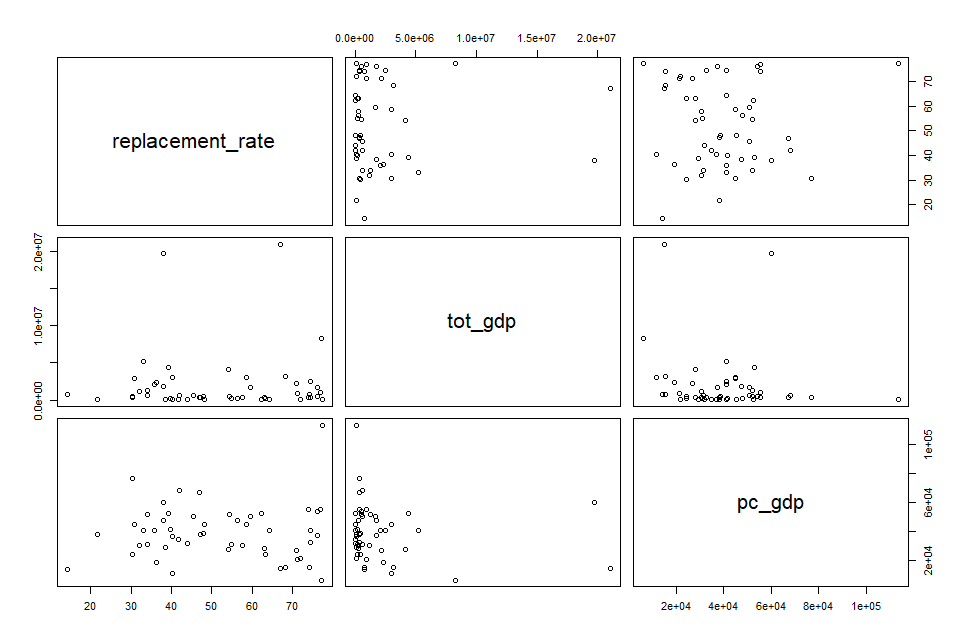There seems no strong relationships. Let's see log10 scatter plot.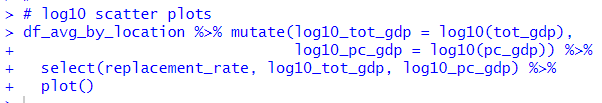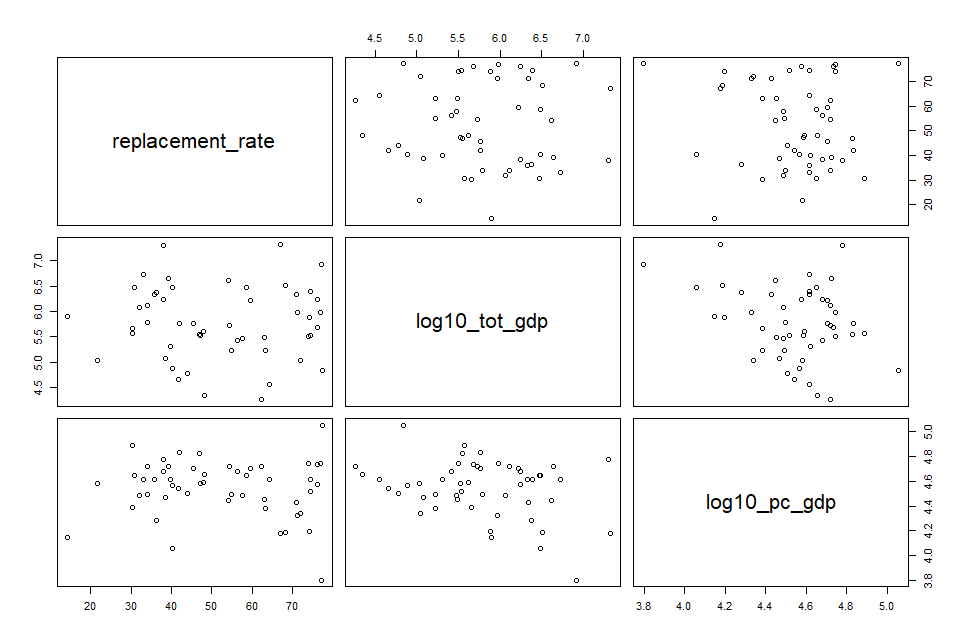Uhm.. there seems no relationships too.

Anyway, let's do linear regression analysis with lm() function.

First, I use tot_gdp and pc_gdp as explanatory variables.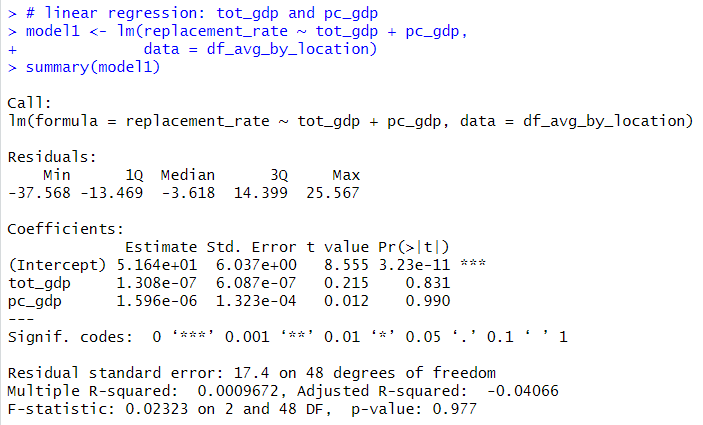p-value is 0.977, so I can say tot_gdp and pc_gdp dosen't matter for replacement_rate.

Next, let's use log10_tot_gdp and log10_pc_gdp as explanatory variables.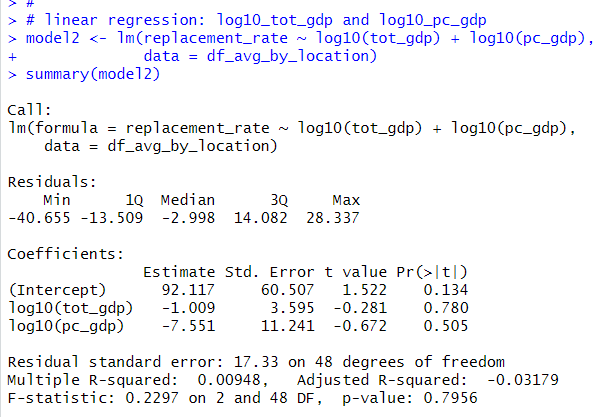p-value is 0.7956, so I can say log10(tot_gdp) and log10(pc_gdp) doesn't matter for replacement rates.

That's it! Thank you!

To read from the first post,